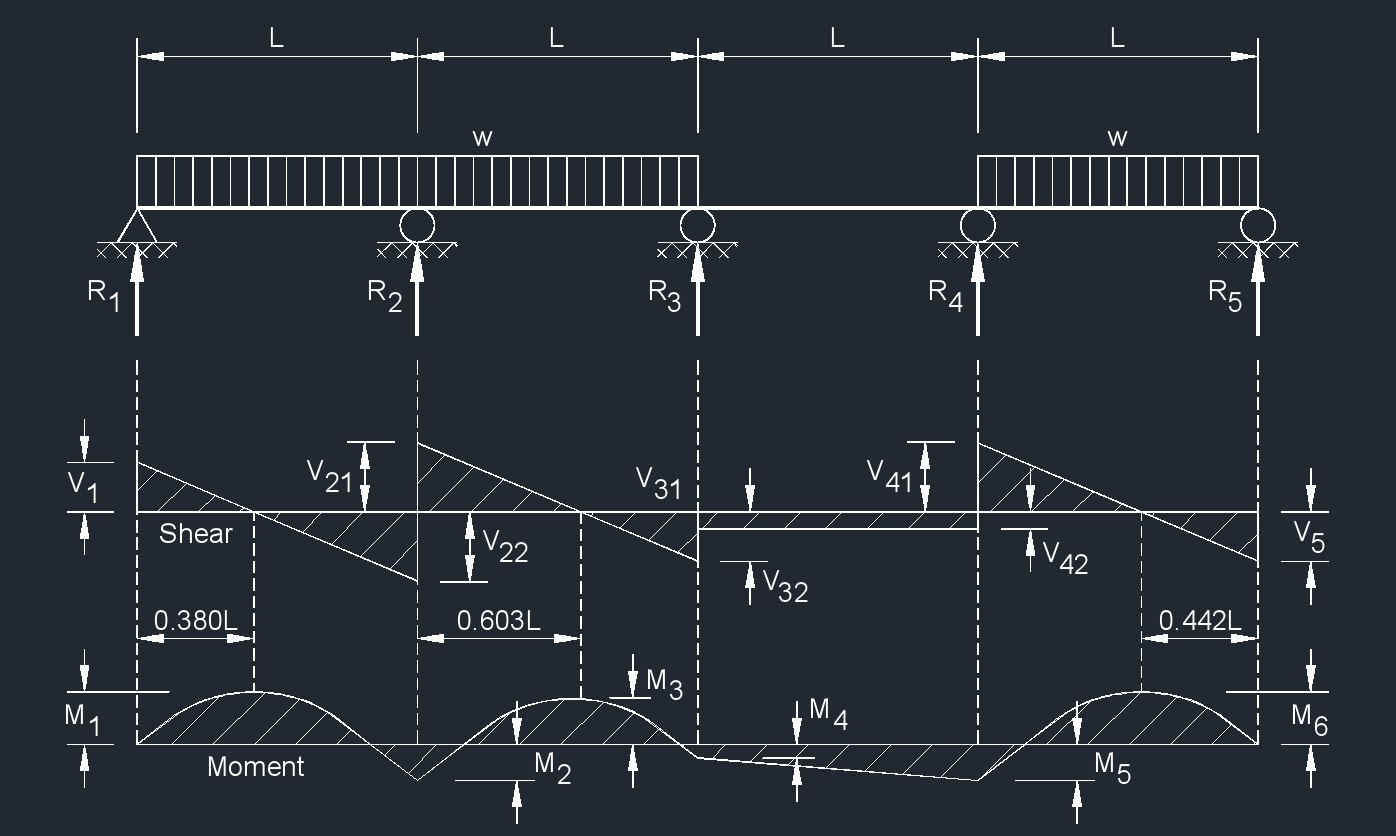# Four Span Continuous Beam - Equal Spans, Uniform Load on Three Spans

Written by Jerry Ratzlaff on . Posted in Structural

###Four Span Continuous Beam - Equal Spans, Uniform Load on Three Spans  Formula

$$\large{ R_1 = V_1 = 0.380wL }$$

$$\large{ R_2 = 1.223wL }$$

$$\large{ R_3 = 0.357wL }$$

$$\large{ R_4 = 0.598wL }$$

$$\large{ R_5 = V_5 = 0.442wL }$$

$$\large{ V_{2_1} = 0.620wL }$$

$$\large{ V_{2_2} = 0.603wL }$$

$$\large{ V_{3_1} = 0.397wL }$$

$$\large{ V_{3_2} = V_{4_1} = 0.040wL }$$

$$\large{ V_{4_2} = 0.558wL }$$

$$\large{ M_1 \; \left( 0.380L \right) \; }$$ from  $$\large{ \left( R_1 \right) = 0.072wL^2 }$$

$$\large{ M_2 \; }$$ at  $$\large{ \left( R_2 \right) \; = -0.1205wL^2 }$$

$$\large{ M_3 \; \left( 0.603L \right) \; }$$ from  $$\large{ \left( R_2 \right) = 0.611wL^2 }$$

$$\large{ M_4 \; }$$ at  $$\large{ \left( R_3 \right) \; = -0.0179wL^2 }$$

$$\large{ M_5 \; }$$ at  $$\large{ \left( R_4 \right) \; = -0.058wL^2 }$$

$$\large{ M_6 \; \left( 0.442L \right) \; }$$ from  $$\large{ \left( R_5 \right) = 0.0977wL^2 }$$

$$\large{ \Delta_{max} \; }$$ at  $$\large{ \left( 0.475L \right) \; }$$ from  $$\large{ \left( R_5 \right) \; = \frac{0.0094wL^4}{\lambda I} }$$

Where:

$$\large{ I }$$ = moment of inertia

$$\large{ L }$$ = span length of the bending member

$$\large{ M }$$ = maximum bending moment

$$\large{ P }$$ = total concentrated load

$$\large{ R }$$ = reaction load at bearing point

$$\large{ V }$$ = shear force

$$\large{ w }$$ = load per unit length

$$\large{ W }$$ = total load from a uniform distribution

$$\large{ x }$$ = horizontal distance from reaction to point on beam

$$\large{ \lambda }$$   (Greek symbol lambda) = modulus of elasticity

$$\large{ \Delta }$$ = deflection or deformation